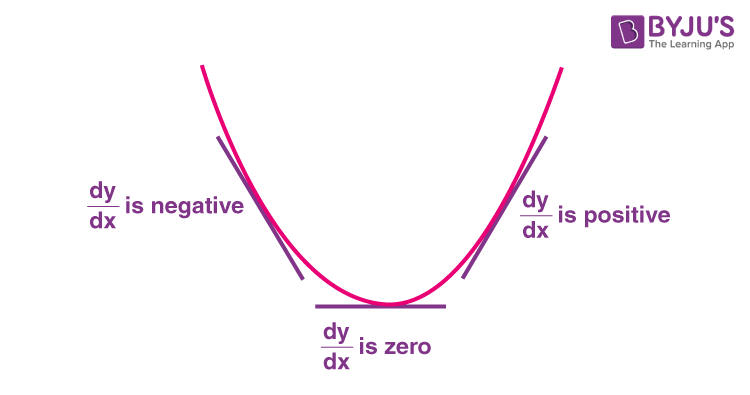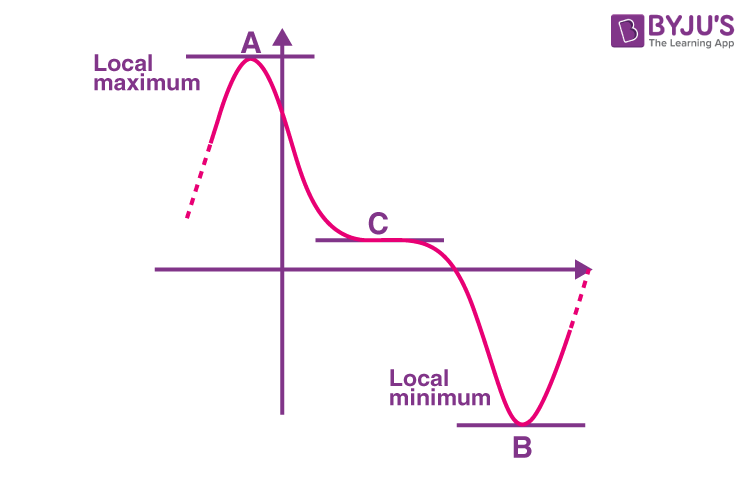Checkout JEE MAINS 2022 Question Paper Analysis : Checkout JEE MAINS 2022 Question Paper Analysis :

# Maxima and Minima in Calculus

Maxima and minima in calculus are found by using the concept of derivatives.  As we know the concept the derivatives gives us the information regarding the gradient/ slope of the function, we locate the points where the gradient is zero and these points are called turning points/stationary points. These are points associated with the largest or smallest values (locally) of the function.

The knowledge of maxima/minima is essential to our day-to-day applied problems. Further, the article also discusses the method of finding the absolute maximum and minimum.

Maxima and minima calculus problems with solutions are given in this article.

## Maxima and Minima Points

Figure for the curve with stationary points is shown below. Thus it can be seen from the figure that before the slope becomes zero it was negative, after it gets zero it becomes positive. It can be said dy/dx is -ve before stationary point dy/dx is +ve after stationary point. Hence it can be said d2 y/dx2 is positive at the stationary point shown below, Therefore it can be said wherever the double derivative is positive it is the point of minima. Vice versa wherever the double derivative is negative is negative is the point of maxima on the curve. This is also known as the second derivative test.Let f be a function defined on an open interval I.
Let f be continuous at a critical point c in I.
If f'(x) does not change sign as x increases through c, then c is neither a point of local maxima nor a point of local minima. Such a point is called a point of inflection.

## Stationary Points vs Turning Points

Stationary points are the points where the slope of the graph becomes zero. In other words the tangent of the function becomes horizontal dy/dx = 0. All the stationary points are given by the figure shown below A,B and C. And the points which the function changes its path if it was going upward it will go downward vice versa i.e. points A and B are turning points since the curve changes its path. But the point C is not turning point although the graph is flat for a short period of time but continues to go down from left to right.## Derivative Tests

Derivative test helps to find the maxima and minima of any function. Usually, first order derivative and second order derivative tests are used. Let us have a look in detail.

### First Order Derivative Test

Let f be the function defined in an open interval I. And f be continuous at critical point c in I such that f’(c) = 0.

1. If f’(x) changes sign from positive to negative as x increases through point c, then c is the point of local maxima. And the f(c) is the maximum value.

2. If f’(x) changes sign from negative to positive as x increases through point c, then c is the point of local minima. And the f(c) is the minimum value.

3. If f’(x) doesn’t change sign as x increases through c, then c is neither a point of local nor a point of local maxima. It will be called the point of inflection.

### Second Derivative Test

Let f be the function defined on an interval I and it is two times differentiable at c.

i. x = c will be point of local maxima if f'(c) = 0 and f”(c)<0. Then f(c) will be having local maximum value.

ii. x = c will be point of local minima if f'(c) = 0 and f”(c) > 0. Then f(c) will be having local minimum value.

iii. When both f'(c) = 0 and f”(c) = 0 the test fails. And that first derivative test will give you the value of local maxima and minima.

### Properties of maxima and minima

1.If f(x) is a continuous function in its domain, then at least one maximum or one minimum should lie between equal values of f(x).

2.Maxima and minima occur alternately. I.e between two minima there is one maxima and vice versa.

3.If f(x) tends to infinity as x tends to a or b and f’(x) = 0 only for one value x i.e.c between a and b , then f(c) is the minimum and the least value.  If f(x) tends to – ∞ as x tends to a or b , then f(c) is the maximum and the highest value.

## Examples on Maxima and Minima

Question 1: Find the turning points of the function y = 4x3 + 12x2 + 12x + 10.

Answer: For turning points dy/dx = 0.

dy/dx = 12x2 + 24x + 12 = 0

=> 3x2 + 6x + 3 = 0

=> (x + 1)(3x + 1) = 0

=> x= -1 and x = (-1)/3

Second Derivative Test:

At x = -1 :

d2 y/dx2 = 24x + 24 = 24(-1) + 24 = -24 + 24 = 0.

Hence x = -1 is the point of inflection. Therefore it is a non turning point.

At x = (-1)/3:

d2y/dx2 = 24x + 24 = 24((-1)/3) + 24 = -8 + 24 = 16.

Hence x = (-1)/3 is a point of minima. Therefore it is a turning point.

Question 2: Find the local maxima and minima of the function f(x) = 3x4 + 4x3 – 12x2 + 12.

For stationary points f'(x) = 0.

f'(x)= 12x3 + 12x2 – 24x = 0

=> 12x(x2 + x – 2) = 0

=> 12x(x – 1)(x + 2) = 0

=> Hence, x = 0, x = 1 and x = -2

Second derivative test:

f”(x) = 36x2 + 24x – 24

f”(x) = 12(3x2 + 2x – 2)

At x = -2

f”(-2) = 12(3(-2)2 + 2(-2) – 2) = 12 (12 – 4 – 2) = 12(6) = 72 > 0

At x = 0

f”(0) = 12(3(0)2 + 2(0) – 2) = 12(-2) = -24 < 0

At x = 1

f”(1) = 12(3(1)2 + 2(1) – 2) = 12(3+2-2) = 12(3) = 36 > 0

Therefore, by the second derivative test x=0 is the point of local maxima while x = -2 and x=1 are the points of local minima.

Question 3: Prove that the radius of the right circular cylinder of greatest curved surface area which can be inscribed in a given cone is half of that of the cone.

Let r and h be the radius and height of the right circular cylinder inscribed in a given cone of radius R and height H. Let S be the curved surface area of cylinder.

S = 2πrh

h = H(R – r)/R

So S = 2πrH(R – r)/R

= (2πH/R)(rR – r2)

Differentiate w.r.t.r

dS/dr = (2πH/R)(R – 2r)

For maxima or minima

dS/dr =0

=> (2πH/R)(R – 2r) = 0

=> R – 2r = 0

=> R = 2r

=> r = R/2

d2S/dr2 = (2πH/R)(0 – 2)

= -4πH/R (negative)

So for r = R/2, S is maximum.

Question 4: A stone is thrown in the air. Its height at any time t is given by h = -5t2+10t+4.

Find its maximum height.

Solution:

Given h = -5t2+10t+4

dh/dt = -10t+10

Now find when dh/dt = 0

dh/dt = 0 ⇒ -10t+10 = 0

⇒ -10t = -10

t = 10/10 = 1

Height at t = 1 is given by h = -5×12+10×1+4

= -5+10+4

= 9

Hence the maximum height is 9 m.

Question 5: Find the maxima and minima for f(x) = 2x3-21x2+36x-15

Solution:

We have f(x) = 2x3-21x2+36x-15

f’(x) = 6x2-42x+36

Now find the points where f’(x) = 0

f’(x) = 0 ⇒ 6x2-42x+36 = 0

⇒  x2-7x+6 = 0

⇒ (x-6)(x-1) = 0

⇒  x = 6 or x = 1 are the possible points of minima or maxima.

Let us test the function at each of these points.

f’’(x) = 12x-42

At x = 1, f’’(1) = 12-42 = -30 <0

Therefore x = 1 is a point of local maximum.

The maximum value is f(1) = 2-21+36-15 = 2

At x= 6, f’’(x) = 12×6-42 = 30> 0

Therefore x = 6 is a point of local minimum.

The local minimum value is f(6) = 2(6)3-21(6)2+36(6)-15

= 2×216-21×36+216-15

= 432-756+216-15 = -123

## Maxima and Minima – Top 12 Must-Do Important JEE Questions### What do you mean by maxima and minima of a function?

Maxima and minima are the maximum and the minimum value of a function respectively, within the given set of ranges.

### How to find maxima and minima algebraically?

We use first order derivative test and second order derivative test to find the maxima and minima of a function.

### Give the steps to find maxima and minima of a function.

Let f(x) be a function. Find the first derivative dy/dx.

Then equate dy/dx to zero and find the critical points.

Then find the second derivative d2y/dx2.

Substitute the critical points in d2y/dx2.

If d2y/dx2 > 0, then that is a point of minima.

If d2y/dx2 < 0, then that is a point of maxima.